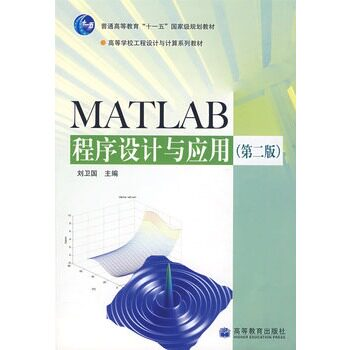#### 正版 MATLAB程序设计与应用（第2版） 刘卫国 高等教育出版社 97

¥8.00

ISBN：9787040188998

ISBN：9787040188998

理论和实践相结合，突出MATLAB在有关学科领域的应用，贴近读者需要。
结构体系得当，脉络清晰，循序渐进，符合认知规律。
内容新，符合技术发展需要。

本书仍保持版的体系结构，全书分为基础篇、应用篇和实验篇。基础篇包括MATLAB系统环境、MATLAB数据及其运算、MATLAB矩阵分析与处理、 MATLAB程序设计、MATLAB图、MATLAB数值计算、MATI。AB符号计算。应用篇包括MATLAB图形用户界面设计、MATLAB Notebook的使用、MATLAB Simulink 仿真软件、MATLAB外部程序接口技术、MATLAB的应用。实验篇和教学内容相配合，包括15个实验，以帮助读者更好地上机操作。

第1章 MATLAB系统环境
1.1 MATLAB概貌
1.1.1 MATLAB的发展
1.1.2 MATLAB的主要功能
1.1.3 MATLAB功能演示
1.2 MATLAB环境的准备
1.2.1 MATLAB的安装
1.2.2 MATLAB的启动与退出
1.3 MATLAB操作界面
1.3.1 主窗口
1.3.2 命令窗口
1.3.3 工作空间窗口
1.3.4 当前目录窗口和搜索路径
1.3.5 命令历史记录窗口
1.3.6 Stalt菜单
1.4 MATLAB帮助系统
1.4.1 帮助命令
1.4.2 帮助窗口
1.4.3 演示系统
习题一
第2章 MATLAB数据及其运算
2.1 MATLAB数据的特点
2.2 变量及其操作
2.2.1 变量与赋值
2.2.2 变量的管理
2.2.3 数据的输出格式
2.3 MATLAB矩阵的表示
2.3.1 矩阵的建立
2.3.2 冒号表达式
2.3.3 矩阵的拆分
2.4 MATLAB数据的运算
2.4.1 算术运算
2.4.2 关系运算
2.4.3 逻辑运算
2.5 字符串
2.6 结构数据和单元数据
2.6.1 结构数据
2.6.2 单元数据
习题二
第3章 MATLAB矩阵分析与处理
3.1 特殊矩阵
3.1.1 通用的特殊矩阵
3.1.2 用于专门学科的特殊矩阵
3.2 矩阵结构变换
3.2.1 对角阵与三角阵
3.2.2 矩阵的转置与旋转
3.3 矩阵求逆与线性方程组求解
3.3.1 矩阵的逆与伪逆
3.3.2 用矩阵求逆方法求解线性方程组
3.4 矩阵求值
3.4.1 方阵的行列式值
3.4.2 矩阵的秩与迹
3.4.3 向量和矩阵的范数
3.4.4 矩阵的条件数
3.5 矩阵的特征值与特征向量
3.6 矩阵的超越函数
习题三
第4章 MATLAB程序设计
4.1 M文件
4.1.1 M文件的分类
4.1.2 M文件的建立与打开
……
第5章 MATLAB绘图
第6章 MATLAB数值计算
第7章 MATLAB符号计算

第8章 MATLAB图形用户界面设计
第9章 MATLABNotebook的使用
第10章 MATLABsimlllink仿真软件
第11章 MATLAB外部程序接口技术
第12章 MATLAB的应用

实验要求
实验一 MATLAB运算基础
实验二 MATLAB矩阵分析与处理
实验三 选择结构程序设计
实验四 循环结构程序设计
实验五 函数文件
实验六 高层绘图操作
实验七 低层绘图操作
实验八 数据处理与多项式计算
实验九 数值微积分与方程数值求解
实验十 符号计算基础与符号微积分
实验十一 级数与方程符号求解
实验十二 菜单与对话框设计
实验十三 Simulink的应用
实验十四 外部程序接口
实验十五 综合实验2016/8/10 11:31:382017/10/11 16:34:072017/10/11 16:33:572017/10/11 16:33:422016/2/7 12:27:572016/1/27 10:33:55